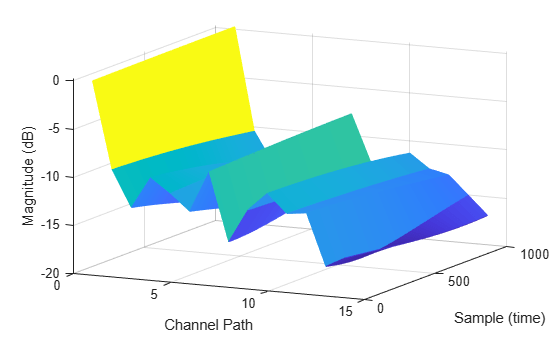# Plot Path Gains for TDL-E Delay Profile with SISO

Plot the path gains of a tapped delay line (TDL) single-input/single-output (SISO) channel using an `nrTDLChannel` System object.

Configure a channel with delay profile TDL-E from TR 38.901 Section 7.7.2. Set the maximum Doppler shift to 70 Hz and enable path gain output.

```tdl = nrTDLChannel; tdl.SampleRate = 500e3; tdl.MaximumDopplerShift = 70; tdl.DelayProfile = 'TDL-E';```

Configure the transmit and receive antenna arrays for SISO operation.

```tdl.NumTransmitAntennas = 1; tdl.NumReceiveAntennas = 1;```

Create a dummy input signal. The length of the input determines the time samples of the generated path gain.

`in = zeros(1000,tdl.NumTransmitAntennas);`

To generate the path gains, call the channel on the input. Plot the results.

```[~, pathGains] = tdl(in); mesh(10*log10(abs(pathGains))); view(26,17); xlabel('Channel Path'); ylabel('Sample (time)'); zlabel('Magnitude (dB)');```